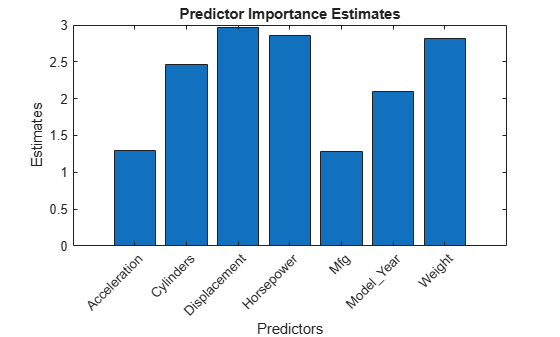Documentation

# predictorImportance

Estimates of predictor importance for regression tree

## Syntax

```imp = predictorImportance(tree) ```

## Description

`imp = predictorImportance(tree)` computes estimates of predictor importance for `tree` by summing changes in the mean squared error due to splits on every predictor and dividing the sum by the number of branch nodes.

## Input Arguments

 `tree` A regression tree created by `fitrtree`, or by the `compact` method.

## Output Arguments

 `imp` A row vector with the same number of elements as the number of predictors (columns) in `tree``.X`. The entries are the estimates of predictor importance, with `0` representing the smallest possible importance.

## Examples

expand all

Estimate the predictor importance for all predictor variables in the data.

Load the `carsmall` data set.

`load carsmall`

Grow a regression tree for `MPG` using `Acceleration`, `Cylinders`, `Displacement`, `Horsepower`, `Model_Year`, and `Weight` as predictors.

```X = [Acceleration Cylinders Displacement Horsepower Model_Year Weight]; tree = fitrtree(X,MPG);```

Estimate the predictor importance for all predictor variables.

`imp = predictorImportance(tree)`
```imp = 1×6 0.0647 0.1068 0.1155 0.1411 0.3348 2.6565 ```

`Weight`, the last predictor, has the most impact on mileage. The predictor with the minimal impact on making predictions is the first variable, which is `Acceleration`.

Estimate the predictor importance for all variables in the data and where the regression tree contains surrogate splits.

Load the `carsmall` data set.

`load carsmall`

Grow a regression tree for `MPG` using `Acceleration`, `Cylinders`, `Displacement`, `Horsepower`, `Model_Year`, and `Weight` as predictors. Specify to identify surrogate splits.

```X = [Acceleration Cylinders Displacement Horsepower Model_Year Weight]; tree = fitrtree(X,MPG,'Surrogate','on');```

Estimate the predictor importance for all predictor variables.

`imp = predictorImportance(tree)`
```imp = 1×6 1.0449 2.4560 2.5570 2.5788 2.0832 2.8938 ```

Comparing `imp` to the results in Estimate Predictor Importance, `Weight` still has the most impact on mileage, but `Cylinders` is the fourth most important predictor.

Load the `carsmall` data set. Consider a model that predicts the mean fuel economy of a car given its acceleration, number of cylinders, engine displacement, horsepower, manufacturer, model year, and weight. Consider `Cylinders`, `Mfg`, and `Model_Year` as categorical variables.

```load carsmall Cylinders = categorical(Cylinders); Mfg = categorical(cellstr(Mfg)); Model_Year = categorical(Model_Year); X = table(Acceleration,Cylinders,Displacement,Horsepower,Mfg,... Model_Year,Weight,MPG);```

Display the number of categories represented in the categorical variables.

`numCylinders = numel(categories(Cylinders))`
```numCylinders = 3 ```
`numMfg = numel(categories(Mfg))`
```numMfg = 28 ```
`numModelYear = numel(categories(Model_Year))`
```numModelYear = 3 ```

Because there are 3 categories only in `Cylinders` and `Model_Year`, the standard CART, predictor-splitting algorithm prefers splitting a continuous predictor over these two variables.

Train a regression tree using the entire data set. To grow unbiased trees, specify usage of the curvature test for splitting predictors. Because there are missing values in the data, specify usage of surrogate splits.

`Mdl = fitrtree(X,'MPG','PredictorSelection','curvature','Surrogate','on');`

Estimate predictor importance values by summing changes in the risk due to splits on every predictor and dividing the sum by the number of branch nodes. Compare the estimates using a bar graph.

```imp = predictorImportance(Mdl); figure; bar(imp); title('Predictor Importance Estimates'); ylabel('Estimates'); xlabel('Predictors'); h = gca; h.XTickLabel = Mdl.PredictorNames; h.XTickLabelRotation = 45; h.TickLabelInterpreter = 'none';```In this case, `Displacement` is the most important predictor, followed by `Horsepower`.